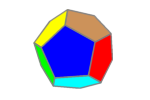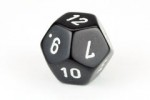# DodecahedronThe dodecahedron is a regular polyhedron made of 12 regular pentagon faces.

A D12 die is often used in certain role playing games.

Since a dodecahedron has 12 sides, you can print out a calendar, one month per face, and have a conversation piece on your desk. (My advice, use heavy paper and score lines before folding.)

The vertex angles are $$cos^{-1} ( \frac{\cos 108}{\cos \frac{108}{2}} )$$ or 121.7174744…°.

To find the dihedral angles, we will start with a dodecahedron with edge length of 1. Let’s take Figure 1 and slice through the drawn lines going across four of the faces. We get a shape similar to some house roofs, with a square base with sides of length Phi (Φ) or $$\frac{1+\sqrt5}{2}$$, as this is the width of a regular pentagon. There are also two triangles and two trapezoids, each with one edge length of Φ and all others 1. In other words, AE=BE=EF=CF=DF=1 and AB=BD=CD=AC=Φ.

Lengths of the pentagon are in Fig. 3, exact representations are not needed at this time.

If we take triangle ABE and “slide” it up to have it meet triangle CDF at point F, shown in red. We will call the points of the new triangle A’, B’, and F, (those are read as A-prime and B-prime). This gives us a rectangular pyramid A’B’CDF.

We know that line EF is 1, so lines AA’ and BB’ are also 1. Lines AC and BD are Φ, so lines A’C and B’D are length Φ-1 or $$\frac{\sqrt5-1}{2}$$, so B’H and HD are each $$\frac{\sqrt5-1}{4}$$.

\begin{align}\left(\frac{\sqrt5-1}{4}\right)^2 + FH^2 &= 1^2 \\ \frac{6-2\sqrt5}{16} + FH^2 &= 1\\ FH^2 &= 1- \frac{6-2\sqrt5}{16} \\ &= \frac{16}{16} – \frac{6-2\sqrt5}{16} \\ &=\frac{10+2\sqrt5}{16} \\ &= \frac{5+\sqrt5}{8} \\ FH &= \sqrt{\frac{5+\sqrt5}{8}} = Cos 18^\circ \end{align}
\begin{align} \cos\angle GFH &= \frac{FG^2 + FH^2 – \Phi^2}{2\cdot FG\cdot FH} \\ &=\frac{\frac{5+\sqrt5}{8} + \frac{5+\sqrt5}{8} – \frac{6+2\sqrt5}{4} }{ 2\cdot \sqrt{\frac{5+\sqrt5}{8}} \cdot \sqrt{\frac{5+\sqrt5}{8}} } \\ &=\frac{\frac{10+2\sqrt5}{8} – \frac{12+4\sqrt5}{8} }{ 2\cdot \frac{5+\sqrt5}{8} } \\ &=\frac{\frac{-2-2\sqrt5}{8} }{ 2\cdot \frac{5+\sqrt5}{8} } \\ &=\frac{-2-2\sqrt5 }{ 2\cdot (5+\sqrt5) } \\ &=\frac{-1-\sqrt5 }{ (5+\sqrt5) } \\ &=\frac{-1-\sqrt5 }{ 5+\sqrt5 }\cdot \frac{-1+\sqrt5}{-1+\sqrt5} \\ &=\frac{1-5+\sqrt5-\sqrt5 }{-5+5-\sqrt5+5\sqrt5} \\ &=\frac{-4}{4\sqrt5}\\ &=\frac{-1}{\sqrt5} \\ \angle GFH &=116.56505\ldots^\circ \end{align}